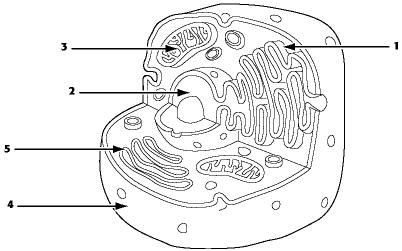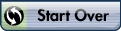# Chapters 1 and 2 PreAP Biology

%CODE1%

### Javascript not enabled

Name:     ID:

Email:

Chapters 1 and 2 PreAP Biology

Multiple Choice
Identify the choice that best completes the statement or answers the question.
1.
Biology is the study of
 a. minerals. c. the weather. b. life. d. energy.
2.
All organisms possess DNA. DNA
 a. creates energy for cells. b. allows sensitivity to environmental stimuli. c. contains information for growth and development. d. captures energy from the sun.
3.
Homeostasis means
 a. a change over long periods of time. b. keeping things the same. c. rapid change. d. the same thing as evolution.
4.
The smallest units that can carry on all the functions of life are called
 a. molecules. c. organelles. b. cells. d. species.
5.
Living things
 a. need energy for life processes. b. have the ability to reproduce. c. are composed of cells. d. All of the above
6.
All living things maintain a balance within their cells and the environment through the process of
 a. growth. c. homeostasis. b. development. d. evolution.
7.
A scientist noticed that in acidic pond water some salamanders developed with curved spines. This was a(n)
 a. hypothesis. c. observation. b. theory. d. control.
8.
The English physician Ronald Ross wanted to try to find the cause of malaria. Based on his observations, Dr. Ross suggested that the Anopheles mosquito might spread malaria from person to person. This suggestion was a
 a. prediction. c. theory. b. hypothesis. d. scientific “truth.”
9.
The English physician Ronald Ross knew that the parasite Plasmodium was always found in the blood of malaria patients. He thought that if the Anopheles mosquitoes were responsible for spreading malaria, then Plasmodium would be found in the mosquitoes. This idea was a
 a. prediction. c. theory. b. hypothesis. d. scientific “truth.”
10.
Scientific hypotheses are most often tested by the process of
 a. communicating. c. experimenting. b. inferring. d. analyzing data.
11.
A hypothesis is
 a. a definite answer to a given problem. b. a testable possible explanation of an observation. c. a proven statement. d. a concluding statement.
12.
A unifying explanation for a broad range of observations is a
 a. hypothesis. c. prediction. b. theory. d. controlled experiment.
13.
A hypothesis that does not explain an observation
 a. is known as an inaccurate forecast. b. often predicts a different observation. c. is rejected. d. None of the above
14.
Scientists usually design experiments
 a. with a good idea of the expected experimental results. b. based on wild guesses. c. in order to develop new laboratory tools. d. All of the above
15.
A scientific theory
 a. is absolutely certain. b. is unchangeable. c. may be revised as new evidence is presented. d. is a controlled experiment.
16.
The word theory used in a scientific sense means
 a. a highly tested, generally accepted principle. b. a guess made with very little knowledge to support it. c. an absolute scientific certainty. d. None of the above
17.
observation : hypothesis ::
 a. theory : observation c. hypothesis : experiment b. guess : hypothesis d. theory : control
18.
Which of the following components of a scientific investigation would benefit from communication between scientists?
 a. observing c. analyzing data b. measuring d. All of the above
19.
Scientists share their research results by
 a. publishing in scientific journals. b. presenting at scientific meetings. c. avoiding conflicts of interest. d. Both a and b
20.
Atoms are composed of
 a. protons with a positive charge. b. neutrons with no charge. c. electrons with a negative charge. d. All of the above
21.
The smallest particle of carbon that can retain the chemical properties of carbon is
 a. a carbon molecule. b. a carbon macromolecule. c. a carbon atom. d. the nucleus of a carbon atom.
22.
A substance that is composed of only one type of atom is called a(n)
 a. nucleus. c. element. b. cell. d. molecule.
23.
All matter in the universe is composed of
 a. cells. c. atoms. b. molecules. d. carbon.
24.
The electrons of an atom
 a. are found in the nucleus along with the protons. b. orbit the nucleus in various energy levels. c. have a positive charge. d. are attracted to the positive charge of neutrons.
25.
Atoms that have gained energy
 a. have protons and neutrons that move farther apart. b. lose neutrons from the nucleus. c. have electrons that move to higher energy levels. d. absorb electrons into the nucleus.
26.
Which of the following states of matter contain(s) particles that are tightly linked together in a definite shape?
 a. solid c. gas b. liquid d. solid and liquid
27.
Because carbon has four electrons in its outer energy level,
 a. it can form bonds with carbon atoms only. b. these atoms are naturally chemically stable. c. it can react with up to four other atoms to form covalent bonds. d. it cannot react with anything other than organic molecules.
28.
The bond formed when two atoms share a pair of electrons is called a
 a. hydrogen bond. c. covalent bond. b. nonpolar bond. d. water bond.
29.
Sharing of electrons between atoms of two or more elements
 a. results in the formation of an ion. b. results in the formation of a compound. c. results in the formation of noble gases. d. results in destabilization of the atoms involved.
30.
An atom that has gained or lost electrons is called a(n)
 a. molecule. c. ion. b. nucleon. d. element.31.
Refer to the graph above. Reaction 1 in the graph
 a. is an energy-storing reaction. b. requires a greater activation energy than reaction 2. c. may use the same initial reactant condition needed to form product Y. d. All of the above
32.
Refer to the graph above. Reaction 3 in the graph
 a. probably occurred in the presence of a catalyst. b. requires a greater activation energy than reaction 2. c. is the same as reaction 1, but faster. d. takes longer than reaction 2.
33.
Refer to the graph above. Which of these statements regarding the graph is true?
 a. Reaction 2 occurs faster than reaction 3 because reaction 2 requires more energy than reaction 3. b. The difference in the graphs shown for reaction 2 and reaction 3 is due to a difference in the activation energy needed for these reactions. c. Reactant X contains more energy at the beginning of the reaction than product Z has after the reaction. d. All of the above
34.
Changing the course or pathway of a chemical reaction so that it requires less activation energy
 a. is a violation of the laws of nature. b. requires higher temperatures than those found within cells. c. occurs only when reactants are quickly added to the reaction mixture. d. is accomplished by the action of catalysts on reactants.
35.
Enzymes
 a. are able to heat up molecules so that they can react. b. provide CO2 for chemical reactions. c. are biological catalysts. d. absorb excess heat so that reactions occur at low temperatures.
36.
When a molecule gains an electron, it has been
 a. oxidized. c. digested. b. reduced. d. inactivated.
37.
Oxidation-reduction reactions are important in organisms because they
 a. allow the passage of energy from molecule to molecule. b. prevent nuclear reactions from occurring. c. allow the creation and destruction of energy. d. None of the above; oxidation-reduction reactions do not occur in living organisms.
38.
The concentration of a solution is
 a. the number of particles of a substance in a solvent. b. the amount of a solvent that is dissolved in a fixed amount of a solution. c. the amount of a solute that is dissolved in a fixed amount of a solution. d. the ratio of solute to solvent in a solution.
39.
A neutral solution has an equal number of
 a. hydrogen and hydronium ions. b. hydroxide and hydronium ions. c. hydrogen and hydroxide ions. d. oxygen and hydrogen ions.
40.
The terms base and alkaline refer to solutions that
 a. contain dissolved sodium hydroxide. b. contain more hydronium ions than hydroxide ions. c. contain more hydroxide ions than hydronium ions. d. contain more hydroxide ions than hydrogen ions.
41.
A solution with a pH of 11 is
 a. acidic. c. neutral. b. alkaline. d. a buffer.
42.
Acidic solutions have a pH that is
 a. less than 7. c. a negative number. b. between 0 and 14. d. more than 7.
43.
Buffers
 a. are of relatively little importance in living things. b. are formed when a large number of hydroxide ions are released in a solution. c. are formed when a large number of hydronium ions are released in a solution. d. tend to prevent great fluctuations in pH.
44.
Atoms become more stable when they form compounds because
 a. they always lose electrons when they form compounds. b. their outer orbitals become filled when they form compounds. c. they always gain electrons when they form compounds. d. their nucleus loses extra protons when they form compounds.
45.
A water molecule is polar because its hydrogen and oxygen atoms
 a. both lose electrons. b. become ions. c. both gain electrons. d. do not share the electrons equally.
46.
Polar molecules such as water have
 a. no negative or positive poles. b. both negative and positive poles. c. only a negative pole. d. only a positive pole.
47.
A molecule that has a partial positive charge on one side and a partial negative charge on the other side is called a
 a. nonpolar molecule. c. charged molecule. b. polar molecule. d. bipolar molecule.
48.
Water is a polar molecule because
 a. it contains two hydrogen atoms for each oxygen atom. b. it has a charge. c. different parts of the molecule have slightly different charges. d. it does not have a charge.
49.
Water molecules break up other polar substances
 a. such as salts. b. because of the uneven charge distribution that exists in water molecules. c. thus freeing ions in these substances for use by the body. d. All of the above
50.
Which of the following characteristics of water is not a result of hydrogen bonding?
 a. adhesive strength b. capillarity c. cohesive strength d. All of the above are a result of hydrogen bonding.